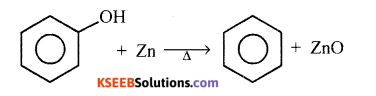# 1st PUC Chemistry Model Question Paper 4 with Answers

Students can Download 1st PUC Chemistry Model Question Paper 4 with Answers, Karnataka 1st PUC Chemistry Model Question Papers with Answers helps you to revise the complete Karnataka State Board Syllabus and score more marks in your examinations.

## Karnataka 1st PUC Chemistry Model Question Paper 4 with Answers

Time: 3.15 Hours
Max Marks: 70

Instruction:

1. The questions paper has five parts A, B, C, D and E. All parts are compulsory.
2. Write balanced chemical equations and draw labeled diagram wherever allowed.
3. Use log tables and simple calculations f necessary (use of scientific calculations is not allowed).

Part – A

I. Answer ALL of the following (each question carries one mark): ( 10 × 1 = 10 )

Question 1.
Give an example for a homogeneous mixture.
Air, Gasoline, Sea water, etc.

Question 2.
Write the ideal gas equation for ‘n’ moles of a gas.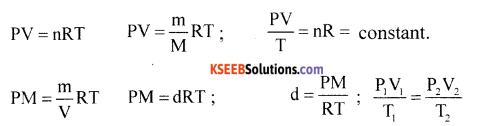Question 3.
What is hydronium ion?
H3O+ is called hydronium ion

Question 4.
Write the IUPAC name of an element with atomic number 107.
Ununnillium.

Question 5.
Identify the element in the compound K2Cr2O7 showing negative oxidation number.
Oxygen.Question 6.
Name the gas liberated when a piece of sodium metal is added into the water.
Hydrogen (H2).

Question 7.
What is Zeolite?
Zeolites are alumino silicates having a three-dimensional cage like structure with a general
formula Mn+x/n [(AlO2)x(SiO2)y]H2O, where M is a cation ion like K+Na+,Ca2+.
These are obtained by replacing some of the silicon atoms by aluminium atoms. The aluminosilicate thus formed acquires negative charge and it is balanced by the cations like
Na+,K+ or Ca2+

Question 8.
Give the composition of water gas.
Water gas [CO + H2] is a mixture of carbon monoxide and hydrogen.

Question 9.
Write the bond line formula for 3-chloro octane.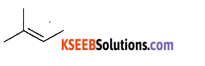Question 10.
Alkanes are not soluble in water. Give reason.
Non-polar and saturated.Part – B

II. Answer any FIVE of the following questions carrying TWO marks: (5 x 2 = 10 )

Question 11.
Calculate the molarity of NaOH in the solution, prepared by dissolving lOg in enough water to form 500mL of the solution. Molar mass of NaOH is 40 g mol-1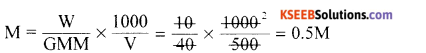Question 12.
Give any two differences between ideal and non-ideal (real) gas.
Ideal Gas

1. Obeys ideal gas equation PV = nRT.
2. Ideal gas does not exist in nature.

Real Gas:

1. Does not obeys the ideal gas equation but obeys van der Waals equation.
2. All gases which exist in nature are real gases.

Question 13.
Mention any two conditions for the combination of atomic orbitals.
The conditions for the combination of atomic orbitals are:
(a) The combining atomic orbitals should have comparable energy.
1s orbital can combine with another Is orbital but not with 2s orbital.

(b) The combining atomic orbitals should have the same symmetry about the molecular axis. For example: 2p orbital can combine with 2s orbital or another 2pz orbital but not with 2px or 2py.
2s cannot combine with 2px or 2py .
(c) The combining atomic orbitals must overlap to the maximum extent.

Question 14.
Complete the following equations:
(i) 4Li + O2 → _____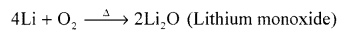(ii) 2Na + O2 → _____
2Na + O2 → Na2O2 (Sodium peroxide)

Question 15.
(a) What is dry ice?
Solid CO2 is called dry ice. It is called dry ice because it directly changes into gaseous state without becoming liquid.(b) Write the formula of basic structural unit of silicates.
Silicates are the compounds in which the anions are present as either discrete SiO^- tetrahedral or a number of such units joined together through comers.

Silicates are basically made up SiO44- tetrahedra (as shown in the figure) in which silicon atom is bonded to four oxygen atoms in a tetrahedral manner. In the figure, big circles indicate oxygen atoms and small circle indicates silicon atoms.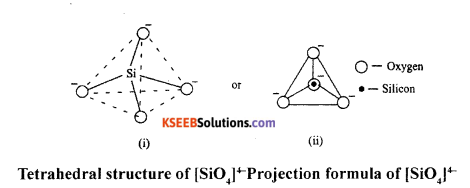Question 16.
Explain Wurtz reaction with a suitable example.
When alkyl halides are heated with sodium metal in ether medium higher alkanes are formed. This reaction is known as Wurtz reaction and employed for the synthesis of higher alkanes containing even number of carbon atoms.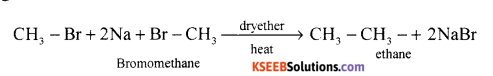Question 17.
What are benzenoids? Give an example.
Aromatic compounds with palanar structure and delocalized n e A above and below the plane of the ring, eg: Benzene, Toluene.

Question 18.
How ozone is formed in the atmosphere? Give equations.
The UV radiations of very short wavelength (< 250 nm) have sufficient energy to cause photo – dissociation of oxygen molecules into oxygen atoms. Highly reactive oxygen atoms combine with oxygen molecules to form ozon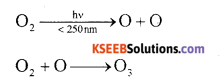Part – C

III. Answer any FIVE of the following questions carrying THREE marks: (5 x 3 = 15 )

Question 19.
(a) Write the general electronic configuration of p-block elements.
General electronic configuration of p-block elements is ns2 np1_6.
Both s and p block together is called representative or normal elements.

(b) How does ionisation enthalpy varies along a period and down the group?
The amount of energy required to remove the most loosely bound electron from an isolated gaseous atom. Ionization energy increases along the period and decreases down the group.

Question 20.
Define the terms (a) Bond angle (b) Bond order (c) Co-valent radius.
(a) Bond angle: It is defined as the “angle between the oribitals containing bonding electron pairs around the central atom in a molecule or in a complex ion”. It is expressed in degrees.
Example: In CO2 the bond angle is 180°. So CO2 has linear shape.
(b) Bond order: It is the number of covalent bonds holding the atoms in the molecule. Example: If the bond is formed by the sharing of two electron pairs, then the bond order is 2. O = O or C = C bond in alkenes.
(c) Co-valent radius: A chemical bond formed by the sharing up of one or more electrons between the atoms.Question 21.
Give any three important postulates of VSEPR theory.
Bonding : The bond formation takes place if there exists impaired electron in the valance shell.
Shape : The geometry or the shape of a molecule depends on the number of valence shell electrons surroundings the central atom.
Repulsion : Electron pair tend to repell one another because electron clouds have similar charge.
Stability : As a result of electron pair repulsion these electron pairs try to stay apart as possible in order to attain minimum energy and maximum stability.
Repulsive interaction : Lane pair with lane pair electrons are having maximum repulsive interaction, bond pair-bond pair electrons are having minimum repulsive interaction. When angle of repulsion decreases angle between the electron pair increases (109°. 28′ less repulsion, 104° more repulsion).

Question 22.
Explain bonding in H2 molecule based on the basis of Molecular orbital theory.
Atomic number of hydrogen is 1 = Is’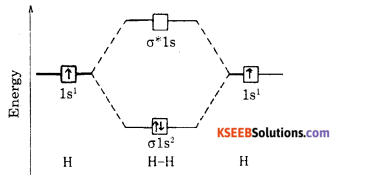(a) Electronic configuration of hydrogen molecule is σ1s2σ*1s0
(b) Bond order – $$\frac{2-0}{2}$$ = 1
(c) Magnet property = diamagnetic.

Question 23.
(a) What is the oxidation number of Cl in KClO,sub>3 ?
+5

(b) Write the separate equations for the oxidation and reduction reactions occuring in the given redox reaction. 2Fe + HCI → FeCL2 + H2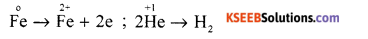Question 24.
Explain the process of softening of temporary hardness by Clark’s method.
Clark’s process: Temporary hardness can be removed by the addition of a calculated amount of lime, where magnesium carbonate or calcium carbonate is precipitated.
Ca(HCO3)2 + Ca(OH)2 → 2CaCO3 +2H2O
Mg(HCO3)2 + Ca(OH)2 → CaCO3 + MgCO3 + 2H3O

Question 25.
(a) Give the composition of plaster of paris and mention one use of it.
Plaster of Paris is CaSO4 + $$\frac{1}{2}$$H2O It is prepared by heating gypsum at 373 K.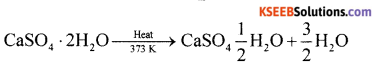Uses:
(i) It is used for manufacture of statues,
(ii) It is used for filling gaps before white washing.

(b) Write any one biological importance of Magnesium.
Biological importance of Mg and Ca are:
(a) Mg ion is present in chlorophyll which is responsible for photosynthesis.
(b) Ca2+ ions are required for maintaining heart beat.
(c) Ca2+ and Mg2+ ions are required for clotting of blood.

Question 26.
(a) Boron shows anamolous behaviour with other elements of the same group. Give reasons.

1. Due to smaller size
2. High ionisation enthalpy
3. High electronegativity.

(b) Name the gas liberated when formic acid is heated with concentrated H2SO4.
Pure carbon monoxide is formed by the dehydration of formic acid with cone. H2SO4.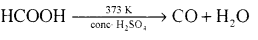Part – D

IV. Answer any FIVE of the following questions carrying FIVE marks: ( 5 x 5 = 25 )

Question 27.
(a) The percentage composition of an organic compound found to contain 39.9% carbon, 6.7% hydrogen and the rest is oxygen. If the molecular mass of the compound is 60 gmol-1. Determine the molecular formula of the compound.
Percentage of oxygen = 100 – (39.9 + 6.7) = 100 – 46.6 = 53.4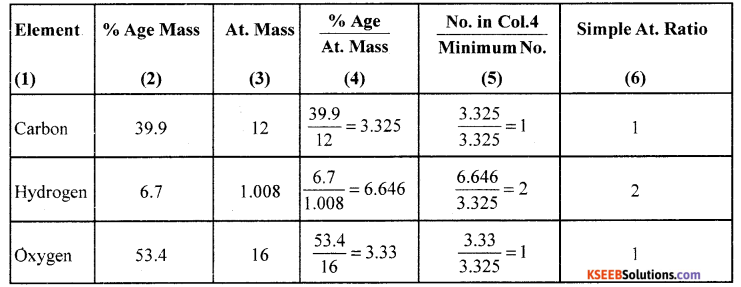∴ Empirical formula = CH2O
Molecular mass = Eq. mass x basicity = 60 x 1 = 60
Empirical formula weight = CH2O = 12 + 2 + 16 = 30
Nows 30n = 60 => n = 2
∴ Molecular formula = (Empirical formula) x n = (CH2O)2 = C2H4O2

Avogadro’s law states that “Equal volumes of all gas under similar conditions of temperature and pressure contain equal number of mole cols.”Question 28.
(a) Give any three postulates of Bohr’s theory of atomic model.
Bohr’s model of an atom, the postulates are:

1. Electrons revolve around the nucleus of an atom in a certain definite path called orbit or stationary state of shell.
2. The shells are having different energy levels denoted as K, L, M, N…
3. As long as the electron remains in an orbit, they neither absorb nor emit energy.
4. The electron can move only in that orbit in which angular momentum is quantized,
i.e., the angular momentum of the electron is an integral multiple of $$\frac{\mathrm{h}}{2 \pi}$$

(b) State Hund’s rule of maximum multiplicity.
Hund’s rule or maximum multiplicity: Electron pairing does not take place until orbitals of same energy are singly occupied.

Question 29.
(a) Name the four quantum numbers and mention what they indicate?
In order to define state energy and location of electron a set of four numbers are used. These numbers are called quantum numbers, (i) Principal quantum number (n). (ii) Azimuthal quantum number (1). (iii) Magnetic quantum number (m). (iv) Spin quantum number (s).

(b) What is node?
The region where probability of finding the electron is zero is called a node.

Question 30.
(a) Write the important postulates of Kinetic theory of gases.

1. Gases are made up of large number of the minute particles.
2. Pressure is exerted by a gas.
3. There is no loss of kinetic theory.
4. Molecules of gas attract on one another.
5. Kinetic energy of the molecule is directly proportional to absolute temperature.
6. Actual volume of the gaseous molecule is very small.
7. Gaseous molecules are always in motion.
8. There is more influence of gravity in the movement of gaseous molecule.

(b) What is Boyle’s temperature?
The temperature at which real gases obey ideal behaviour for appreciable range of pressure is called Boyle’s temperature (or) Boyle’s point.

(c) Falling liquid droplets are spherical. Give reason.
Due to surface tension.

Question 31.
(a) Calculate the standard enthalpy of formation of benzene from the following data.
C6H6 + O2 + $$\frac{15}{2}$$O2  → 6CO2 + 3H2O(l); ∆H= – 3264KJ
C(s) + O2(g)  → CO2(g); ∆H = -393.5KJ
H2 + $$\frac{1}{2}$$O2 → H2O(l); ∆H = -285.9KJ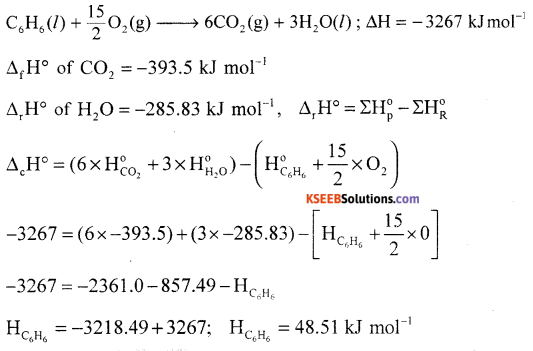(b) What is entropy?
Measure of disordemess of a system.Question 32.
(a) Calculate the total work done when one mole of a gas expands isothermally and reversibly from an intial volume of 10dm3 to a final volume of 20dm3 at 298K.
T = 27°C = 27 + 273 = 300K , V1 = 10 dm3 V2 = 20 dm3 , R = 8.314 J/K/mol
w = -2.303 nRTlog $$\left(\frac{v_{2}}{v_{1}}\right)$$
w = -2.303 x 1 x 8.314 x 300 x log $$\left(\frac{20}{10}\right)$$= -2.303 x 8.314 x 300 x log2
= -2.303×8.314x300x0.3010 = -1729 joule = -1.723 kJ.

(b) What is an intensive property? Give an example.
Intensive property of a system is that property of the system which does not depend on the quantity of the substance present in the system. E.g; Density, Viscosity.

Question 33.
(a) For the hydrolysis of sucrose the equilibrium constant Kc is x x 10-3 at 300K calculate ∆G° at 300K?
Given Kc = 2 x 10-3
T = 300K; ∆G° = ?
We know that ∆G° = -2.303 RT log Kc = -2.303 x 8.314 x 300 x 2 x 10-3
= -2.303 x 8.314 x 3 x 2 = -11.488 J/mol.

(b) Explain common ion effect with suitable example.
Suppression in the degree of dissociation of a weak electrolyte by the addition of a common ion is called common ion effect.

Question 34.
(a) Calculate the pOH of a solution obtained when 0.05 mol NH4Cl is added and dissolved , in 0.025M ammonia solution. (Given Kb for ammonia = 1.77 x 10-5).
pKb = -logKb = -logl.8 x 10-5 = -(5.2553) = 4.7447
pOH = pKb + log $$\frac{[\text { salt }]}{[\text { base }]}$$ = 4.7447 + log $$\frac{0.05}{0.12}$$ = 4.74 – 0.38 = 4.36
∴ pH = 14 – pOH= 14 – 4.36 = 9.64

(b) What is solubility product?
It is the product of the molar concentration of ions of the saturated solution of a sparingly soluble solution of the salt and the coefficients are raised to their powers at constant temperature.

Part – E

V. Answer any TWO of the following questions carrying FIVE marks: ( 2 x 5 = 10 )

Question 35.
(a) Write the principles involved in the estimation of
(i) Halogens (ii) Sulphur present in an organic compound by Carius method.
(i) Halogens: When an organic compound containing halogen (Cl, Br or 1) is heated in a sealed tube with fuming nitric acid and excess of silver chloride, silver halide is formed from the mass of silver halide obtained, the percentage of the halogen can be calculated.

Procedure: In a hard glass tube (Carius tube), 5 ml of fuming HNO3 and 2 to 2.5 g AgNO3 are taken. A small narrow weighing tube, containing a small amount (nearly 0.15 -0.2g) of accurately weighed organic compound, is introduced in the Carius tube in such a way that nitric acid does not enter the weighing tube. The Carius tube is now sealed and heated in a furnace at 300°C for about six hours.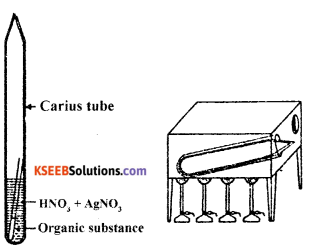The tube is then cooled and its narrow end is cut off and the contents are completely transferred to a beaker by washing with water. The precipitate of silver halide formed is filtered through a weighed sintered glass crucible. It is washed, dried and weighed.

Observation and calculation:
(i) Mass of organic compound taken = W1g
(ii) Mass of silver halide obtained = w2g
(a) For chlorine: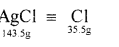143.5g of AgCl contains 35.5 g of chlorine.
w2g of AgCl will contain $$\frac{35 \cdot 5 \times w_{2}}{143 \cdot 5}$$ of chlorine
∴ % Cl2 = $$\frac{35 \cdot 5 \times w_{2}}{143 \cdot 5} \times \frac{100}{w_{1}}$$

(b) For bromine: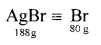188 g of AgBr contains 80 g of bromine
w2 of AgBr will contain $$\frac{980 \times w_{2}}{188}$$ g of bromine

(ii) Sulphur Present in an organic compound by carius method:
Principle: An organic compound containing sulphur to heated in Carius tube with fuming nitric acid or sodium peroxide. Sulphur present is oxidised to sulphuric acid. It is then precipitated as burium sulphate by adding excess of barium chloride solution. The precipitate formed is filtred, washed, dried and weighed. The % of S is calculated from the mass of barium sulphate.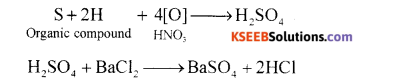Calculations: Mass of organic substance = ‘m’
Mass of barium sulphate formed = ‘x’ g.
Molecular mass of barium sulphate = 233 g.(b) Name the element estimated by Kjeldahl’s method.
Nitrogen.Question 36.
(a) Describe the estimation of Carbon and Hydrogen by Liebig’s method.

Principle: A known mass of an organic compound is strongly heated with dry cupric oxide (CuO), when carbon and hydrogen are quantitatively oxidized to CO2 and H2O respectively. The masses of CO2 and H2O thus formed are determined. From this, the percentages of carbon and hydrogen can be calculated.
Procedure : Pure and dry oxygen is passed through the entire assembly of the apparatus (Figure) till the CO2 and moisture is completely removed.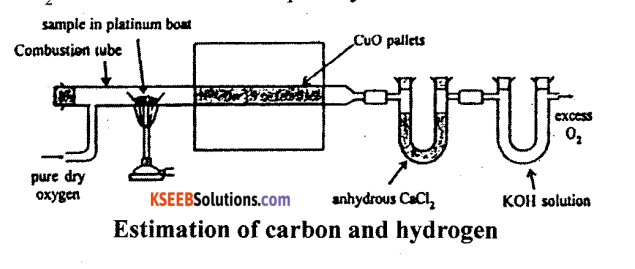A boat containing weighed organic substances is introduced inside from one end of the combustion tube by opening it for a while. The tube is now strongly heated till the whole of the organic compound is burnt up. The flow of oxygen is continued to drive CO2 and water vapours completely to the U-tubes. The apparatus is cooled and the U-tubes are weighed separately.

Observation and calculations:

1. Mass of organic compound taken = w.g.
2. Mass of water produced = x g (Increase in mass of CaCl2 tube).
3. Mass of carbon dioxide produced = y g (Increase in mass of KOH tube).

To determine % of carbon:
Molar mass of CO2 = 44g mol-1
Now, 44g of CO2 = contains 12 g of C
y g of CO2 will contain of $$\frac{12 y}{44}$$ f of C
This amount of carbon was present in w. g. of the substance
∴ % C = $$\frac{12 y}{44} \times \frac{100}{w}$$

To determine % of Hydrogen
Molar mass of water = 18 g mol-1
Now, 18 g of H2O contains 2 g of H2O
∴ x g of H2O will contain $$\frac{2 x}{18}$$ g of H2O
This amount of hydrogen was present in weight of substance.
∴ H2 = $$\frac{2 x}{18} \times \frac{100}{w}$$

(b) What is Cracking?
The decomposition of higher alkane into a mixture of lower alkanes alkenes, etc. by the applicationQuestion 37.
(a) Explain the mechanism of chlorination of Methane.
Mechanism of chlorination of methane involves three types:

Step 1: Initiation: Chlorine absorbs energy and undergoes homolysis to give chlorine free radicals.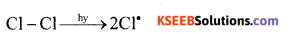Step 2: Propagation: Chlorine free radical reacts with methane to give methyl free radical.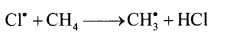The methyl free radical reacts with chlorine to form methyl chloride and chlorine free radical.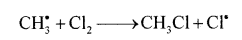Step 3: Termination: Free radials combine to form stable products.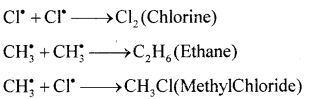(b) Name the product formed when phenol vapours are passed over heated zinc dust. Write the chemical equation.